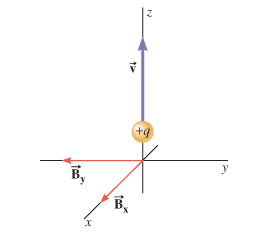# Magnetic field in 3 dimensions

## Homework Statement

One component of a magnetic field has a magnitude of 0.0431 T and points along the +x axis, while the other component has a magnitude of 0.0686 T and points along the -y axis. A particle carrying a charge of +1.70 × 10-5 C is moving along the +z axis at a speed of 4.24 × 103 m/s. (a) Find the magnitude of the net magnetic force that acts on the particle. (b)Determine the angle that the net force makes with respect to the +x axis.

## Homework EquationsF= qvBsinθ

## The Attempt at a Solution

I don't know how to get Bnet, I was thinking it would be by using the pythagorean theorem.

tnich
Homework Helper

## Homework Statement

One component of a magnetic field has a magnitude of 0.0431 T and points along the +x axis, while the other component has a magnitude of 0.0686 T and points along the -y axis. A particle carrying a charge of +1.70 × 10-5 C is moving along the +z axis at a speed of 4.24 × 103 m/s. (a) Find the magnitude of the net magnetic force that acts on the particle. (b)Determine the angle that the net force makes with respect to the +x axis.

## Homework Equations

View attachment 228631
F= qvBsinθ

## The Attempt at a Solution

I don't know how to get Bnet, I was thinking it would be by using the pythagorean theorem.
That should work.

Thank you